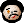# 2019-05-11 Toggling modeline clock display

As I mentioned a lot of times, I use Org-mode clocking all the time. Among others, I integrated it with two external services, I have a few dedicated keybindings to it in my Org-mode hydra. It’s no wonder that I work hard to make clocking as smooth as possible.

I used to have the variable `org-clock-mode-line-total` set to `'today`. This means that the clock info in my modeline reflects the time I spent on this particular task, well, today. (This fits nicely in my Beeminder workflow, where I set daily goals for various stuff, measured in minutes).

However, I’m also working on my productivity, and I was toying with the idea of using the famous pomodoro technique. The main idea is that you work in 25-minute intervals with 5-minute breaks.

While I do not like the rigidity of that scheme, the idea appeals to me. There are a few Emacs-based solutions (and even one or two Org-mode ones), but none of them really resonates with me. I will definitely look into integrating some kind of timeboxing technique into my workflow in the future, but for now, I settled for a minimalist solution.

The idea is that both `'today` and `'current` are reasonable values of `org-clock-mode-line-total` for me, and hence I’d like to be able to switch between them seamlessly. This way, I could either easily see how much time I spent on the currently clocked task in this particular “timebox”, or how much I spent on it today. (Having that, I decided that seeing how much I spent on it altogether can also be useful.) Since I already have my Org-mode related hydra, I decided to add one more command to it: `toggle-org-clock-mode-line-total-setting`. The idea is to be able to cycle between various settings of `org-clock-mode-line-total` with a keystroke.

So, I started with this:

```(setq org-clock-mode-line-total-settings
'((current . "time spent in this chunk on the current task")
(today . "time spent today on the current task")
(all . "total time spent on the current task")))
(setq org-clock-mode-line-total-setting-number 0)
```

This is a very rudimentary implementation of a “ring”. A ring is just like a list, only when we want to go past the last element, we get at the first again. Actually, Emacs provides a “ring” package which implements such a data structure efficiently, but I decided that it would be an overkill for just three elements – here, the simplicity of the code won over the efficiency. (In fact, after looking into the “ring” package, I’m no longer sure whether my code is really that simple… I had to do the modulo wrapping by hand, for instance – Emacs’ “ring” does that for me.)

Now, let us implement the actual cycling. This proved (quite unexpectedly) to be the hard part, since just toggling `org-clock-mode-line-total` did not do the trick – somehow, I had to make Emacs recount the time of the current clocking item elapsed from the first clocking entry or just for today or the current entry.

```(defun toggle-org-clock-mode-line-total-setting (setting-number)
"Toggle between org-clock-mode-line-total settings.
With a numeric argument, use setting SETTING-NUMBER."
(interactive "P")
(if (numberp setting-number)
(setq org-clock-mode-line-total-setting-number
(mod setting-number (length org-clock-mode-line-total-settings)))
(setq org-clock-mode-line-total-setting-number
(mod (1+ org-clock-mode-line-total-setting-number)
(length org-clock-mode-line-total-settings))))
(let ((org-clock-mode-line-total-setting (nth org-clock-mode-line-total-setting-number
org-clock-mode-line-total-settings)))
(setq org-clock-mode-line-total (car org-clock-mode-line-total-setting))
(when (org-clocking-p)
(setq org-clock-total-time
(with-current-buffer (marker-buffer org-clock-hd-marker)
(save-excursion (goto-char org-clock-hd-marker)
(org-clock-sum-current-item
(org-clock-get-sum-start)))))
(org-clock-update-mode-line))
(message "Modeline shows %s."
(cdr org-clock-mode-line-total-setting))))
```

The tricky thing is the latter part of the above code. Just changing the value of `org-clock-mode-line-total` in the middle of clocking does not have any effect – even if we actually call `org-clock-update-mode-line`. What we need to do is to call `org-clock-get-sum-start`, which (as Nick Dokos pointed to me) is the only place in the Org-mode sources where our variable is used. Mimicking its use in `org-clock-in`, I set the global variable `org-clock-total-time` and only then call `org-clock-update-mode-line`. This function calls `org-clock-get-clock-string`, which calls `org-clock-get-clocked-time`, which uses `org-clock-total-time` and adds the currently clocked time to its value.

Elementary, my dear Watson.

The moral of this story is that while Org-mode source code is sometimes a big mess, you can still do nice things if you are patient enough and can use grep (or ask wise people than can use it).